# Electric Field / Electric Potentiel / Electric Current

1) A 5.0-C charge is 10 m from a small test charge. What is the magnitude of the electric field at the location of the test charge?

2)Two parallel plates, separated by 0.20 m, are connected to a 12-V battery. An electron released from rest at a location 0.10 m from the negative plate. When theelectron arrives at a distance 0.050 m from the positive plate, how much kinetic energy does the electron gain?

3)A 4.0-g object carries a charge of 20 µC. The object is accelerated from rest through a potential difference, and afterward the ball is moving at 2.0 m/s. What isthe magnitude of the potential difference?

4)A point charge of +Q is placed at the center of a square. When a second point charge of -Q is placed at one of the square's corners, it is observed that anelectrostatic force of 2.0 N acts on the positive charge at the square's center. Now, identical charges of -Q are placed at the other three corners of the square. Whatis the magnitude of the net electrostatic force acting on the positive charge at the center of the square?

5)The absolute potential at the center of a square is 3.0 V when a charge of +Q is located at one of the square's corners. What is the absolute potential at thesquare's center when a second charge of -Q is placed at one of the remaining corners?

6)Three identical charges of 3.0 µC are placed at the vertices of an equilateral triangle which measures 30 cm on a side. What is the magnitude of the electrostaticforce which acts on any one of the charges?

7)Three point charges are placed on the x-axis. A charge of +2.0 µC is placed at the origin, -2.0 µC to the right at x = 50 cm, and +4.0 µC at the 100 cm mark. Whatare the magnitude and direction of the electrostatic force which acts on the charge at the origin?
Answer 0.14 N right or 0.072 N left or 0.14 N left or 0.072 N right.

8) Q1 = 6.0 nC is at (0.30 m, 0); Q2 = -1.0 nC is at (0, 0.10 m); Q3 = 5.0 nC is at (0, 0). What is the direction of the net force on the 5.0 nC charge?

This Homework Help Question: "Electric Field / Electric Potentiel / Electric Current" No answers yet.

We need 10 more requests to produce the answer to this homework help question. Share with your friends to get the answer faster!

0 /10 have requested the answer to this homework help question.

Once 10 people have made a request, the answer to this question will be available in 1-2 days.
All students who have requested the answer will be notified once they are available.

#### Earn Coin

Coins can be redeemed for fabulous gifts.

Similar Homework Help Questions
• ### electric fields and potentials

A point charge of +3Q is placed at the center of a square. When a second point charge of -Q is placed at one of the square's corners, it is observed that anelectrostatic force of 4 N acts on the positive charge at the square's center. Now, identical charges of -Q are placed at the other three corners of the square. Whatis the magnitude of the net electrostatic force acting on the positive charge at the center of the square

• ### What is the magnitude of the net electrostatic force acting on the positive charge at the center...

A point charge of +4Q is placed at the center of a square. When a second point charge of -Q is placed at one of the square's corners, it is observed that anelectrostatic force of 1 N acts on the positive charge at the square's center. Now, identical charges of -Q are placed at the other three corners of the square. Whatis the magnitude of the net electrostatic force acting on the positive charge at the center of the square?answer...

• ### (Figure 1) shows five electric charges. Four charges with the magnitude of the charge 2.0 nC...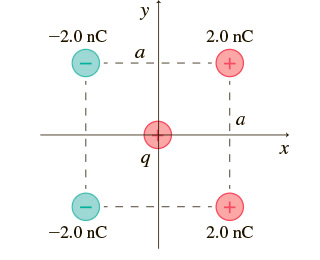(Figure 1) shows five electric charges. Four charges with the magnitude of the charge 2.0 nC form a square with the size a = 3.0 cm . Positive charge with the magnitude of q = 7.0 nC is placed in the center of the square. What is the direction of the force on the 7.0 nC charge in the middle of the figure due to the four other charges? What is the direction of the force on the 7.0 nC...

• ### (Figure 1) shows five electric charges. Four charges with the magnitude of the charge 2.0 nC...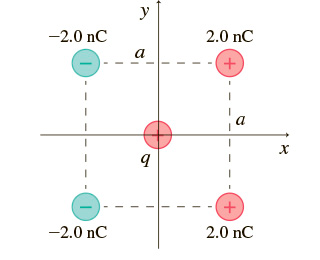(Figure 1) shows five electric charges. Four charges with the magnitude of the charge 2.0 nC form a square with the size a = 1.0 cm . Positive charge with the magnitude of q = 6.5 nC is placed in the center of the square. 1. What is the magnitude of the force on the 6.5 nC charge in the middle of the figure due to the four other charges? 2. What is the direction of the force on the...

• ### Answer ALL problems using the bubble sheet given(will be distributed to class on MONDAY). Please SHOW...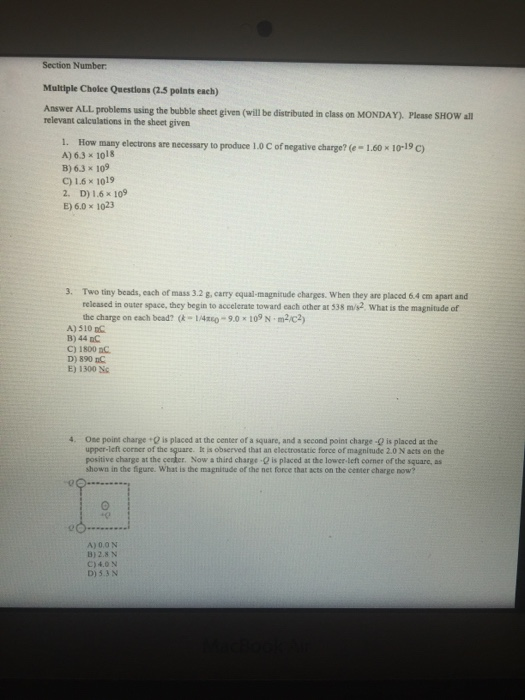Answer ALL problems using the bubble sheet given(will be distributed to class on MONDAY). Please SHOW all relevant calculations in the sheet given How many electrons are necessary to produce 1.0C of negative charge?(e=1.60times10^-19C) 6.5times10^18 6.3times10^19 1.6times10^19 1.6times10^9 6.0times10^23 Two tiny beads, each of mass 3.2g, carry equal-magnitude charges. When they are placed 6.4 cm apart and released in outer space, they begin to accelerate toward each other at 538 m/s^2. What is the magnitude of he charge on each...

• ### Part A (Figure 1) shows five electric charges Four charges with the magnitude of the charge...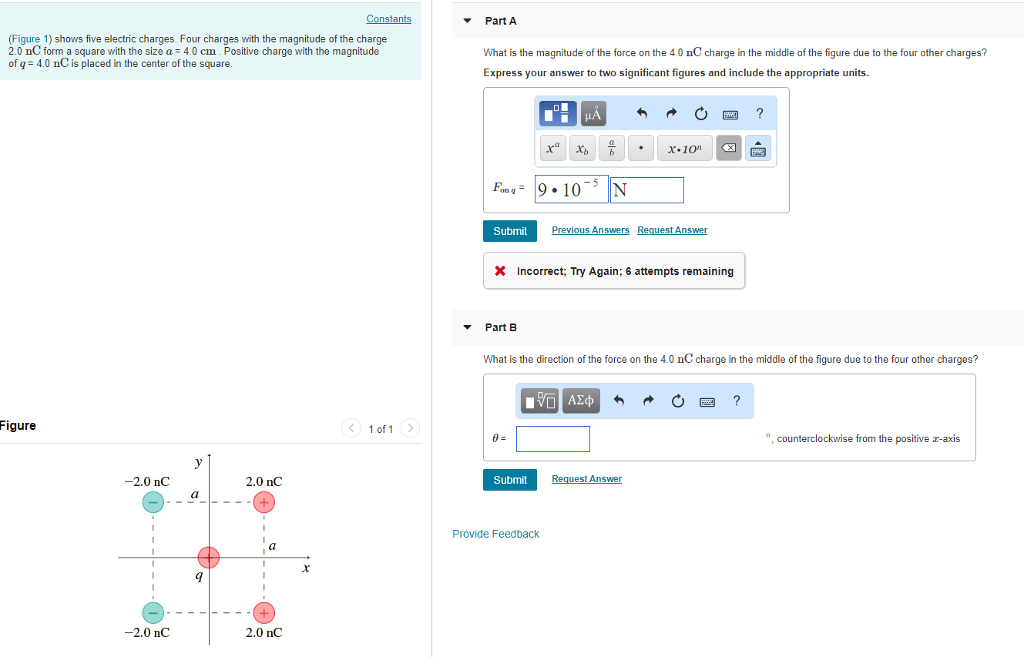Part A (Figure 1) shows five electric charges Four charges with the magnitude of the charge 2.0 nC form a square with the size a 4.0 cm. Positive charge with the magnitude of q-4.0 nC is placed in the center of the square. What is the magnitude of the force on the 4.0 nC charge in the middle of the figure due to the four other charges? Express your answer to two significant figures and include the appropriate units. Submit...

• ### Review Constants Part A (Figure 1) shows five electric charges. Four charges with the magnitude of...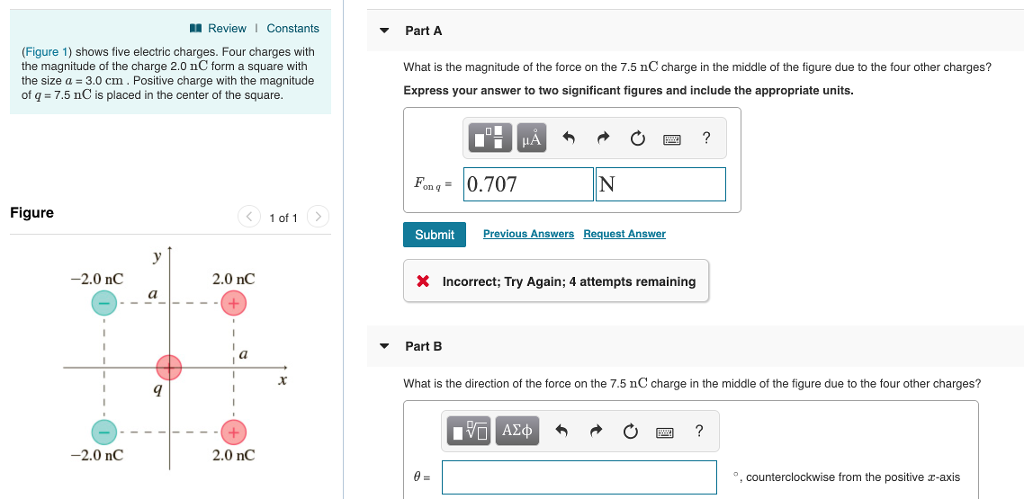Review Constants Part A (Figure 1) shows five electric charges. Four charges with the magnitude of the charge 2.0 nC form a square with the size a-3.0 cm. Positive charge with the magnitude of q = 7.5 nC is placed in the center of the square What is the magnitude of the force on the 7.5 nC charge in the middle of the figure due to the four other charges? Express your answer to two significant figures and include the...

• ### Four point-like charges are placed as shown in the figure, three of them are at the...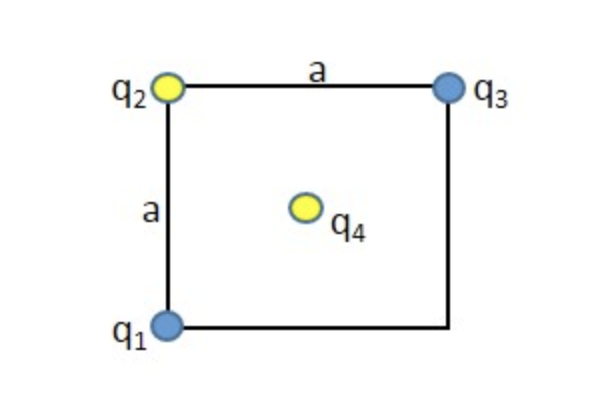Four point-like charges are placed as shown in the figure, three of them are at the corners and one at the center of a square, 40.0 cm on each side. Find the magnitude of the net electrostatic force exerted on the point charge q 4 . Let q 1 = q 3 = +27.0 µC, q 2 = −26.0 µC, and q 4 = −36.0 µC. 42 93

• ### Four point-like charges are placed as shown in the figure, three of them are at the...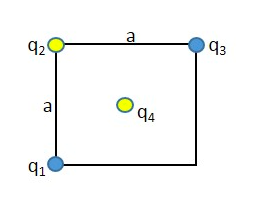Four point-like charges are placed as shown in the figure, three of them are at the corners and one at the center of a square, 36.0 cm on each side. Find the magnitude of the net electrostatic force exerted on the point charge q 4 . Let q 1 = q 3 = +14.0 µC, q 2 = −26.0 µC, and q 4 = −52.0 µC. 42 43 4

• ### Four point-like charges are placed as shown in the figure, three of them are at the...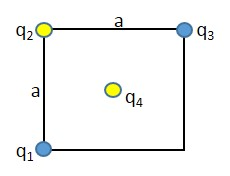Four point-like charges are placed as shown in the figure, three of them are at the corners and one at the center of a square, 20.0 cm on each side. Find the magnitude of the net electrostatic force exerted on the point charge q4. Let q1 = q3 = +19.0 µC, q2 = ?37.0 µC, and q4 = ?49.0 µC. Q29 q3 Qa q